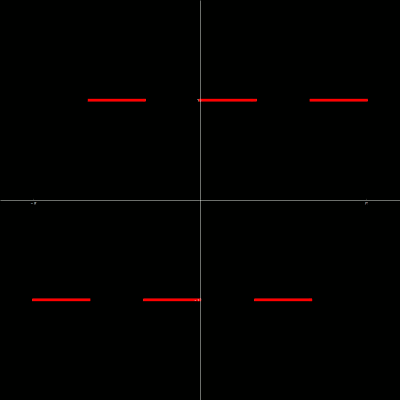M A T H 2 1 B
Mathematics Math21b Spring 2017
Linear Algebra and Differential Equations
Linear algebra Math21b, Spring 2017
 The exams page has now the exam and a solution draft. The exhibits page has more entries like the Determinant sticker or Pascal matrices which appeared in the Final exam [PDF]. The following handouts are crucial for the last part of the course The pendulum (animated below in Javascript) is simplified from a double pendulum. It is a periodically driven pendulum with friction x''=-sin(x)+ c x' + a sin(t) a system which is already chaotic in certain domains.